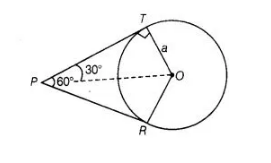# If angle between two tangents drawn

Question:

If angle between two tangents drawn from a point P to a circle of radius a and centre 0 is 60°, then OP = a√3.

Solution:

True

From point $P$, two tangents are drawn.Given,  $O T=a$

Also line $O P$ bisects the $\angle R P T$.

$\angle T P O=\angle R P O=30^{\circ}$

Also, $O T \perp P T$

In right angled $\triangle O T P$,

$\sin 30^{\circ}=\frac{O T}{O P}$

$\Rightarrow$ $\frac{1}{2}=\frac{a}{O P}$

$\Rightarrow$ $O P=2 a$